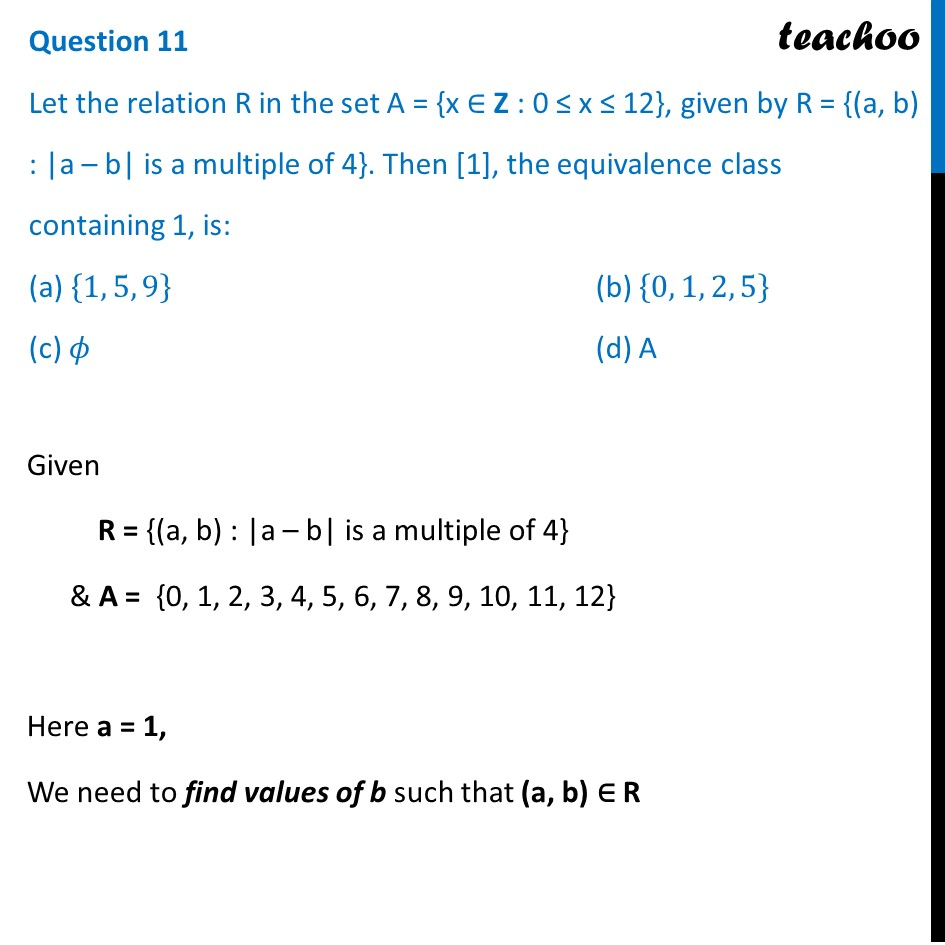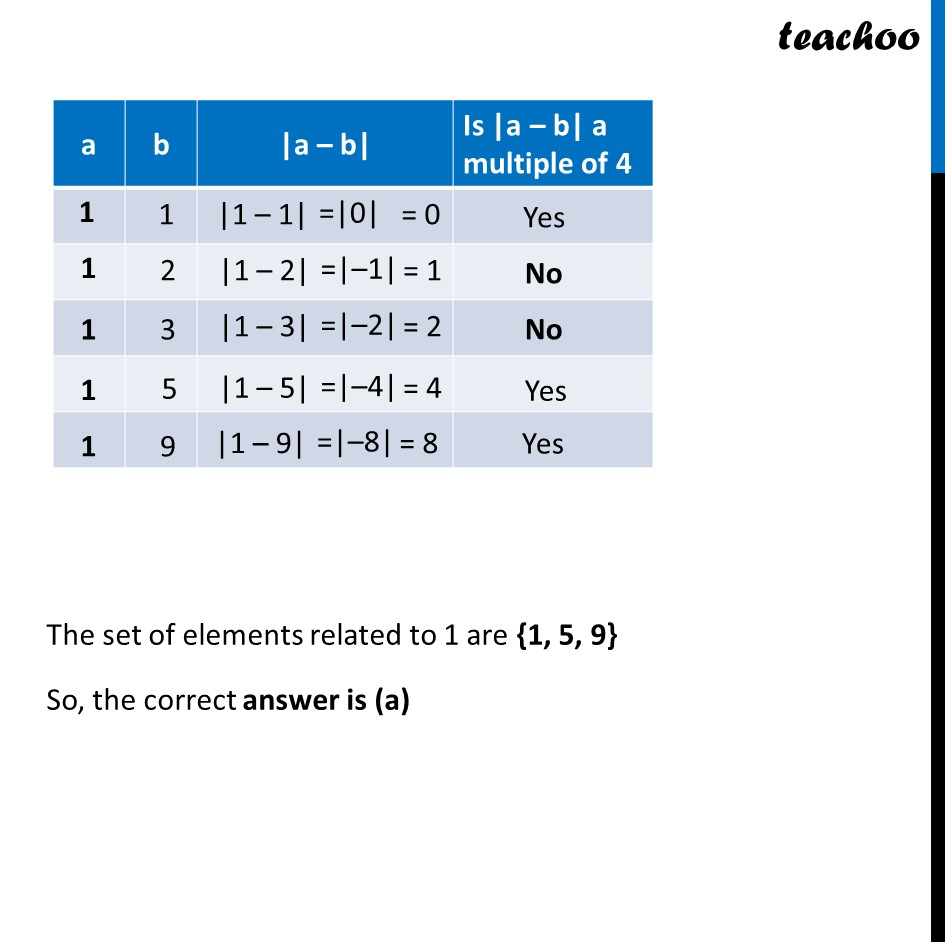CBSE Class 12 Sample Paper for 2022 Boards (MCQ Based - for Term 1)

Class 12
Solutions of Sample Papers and Past Year Papers - for Class 12 Boards

## (c) ϕ                    (d) A

This question is inspired from Ex 1.1, 9 (i) - Chapter 1 Class 12 - Relation and FunctionsLearn in your speed, with individual attention - Teachoo Maths 1-on-1 Class

### Transcript

Question 11 Let the relation R in the set A = {x ∈ Z : 0 ≤ x ≤ 12}, given by R = {(a, b) : |a – b| is a multiple of 4}. Then , the equivalence class containing 1, is: (a) {1, 5, 9} (b) {0, 1, 2, 5} (c) 𝜙 (d) A Given R = {(a, b) : |a – b| is a multiple of 4} & A = {0, 1, 2, 3, 4, 5, 6, 7, 8, 9, 10, 11, 12} Here a = 1, We need to find values of b such that (a, b) ∈ R The set of elements related to 1 are {1, 5, 9} So, the correct answer is (a)